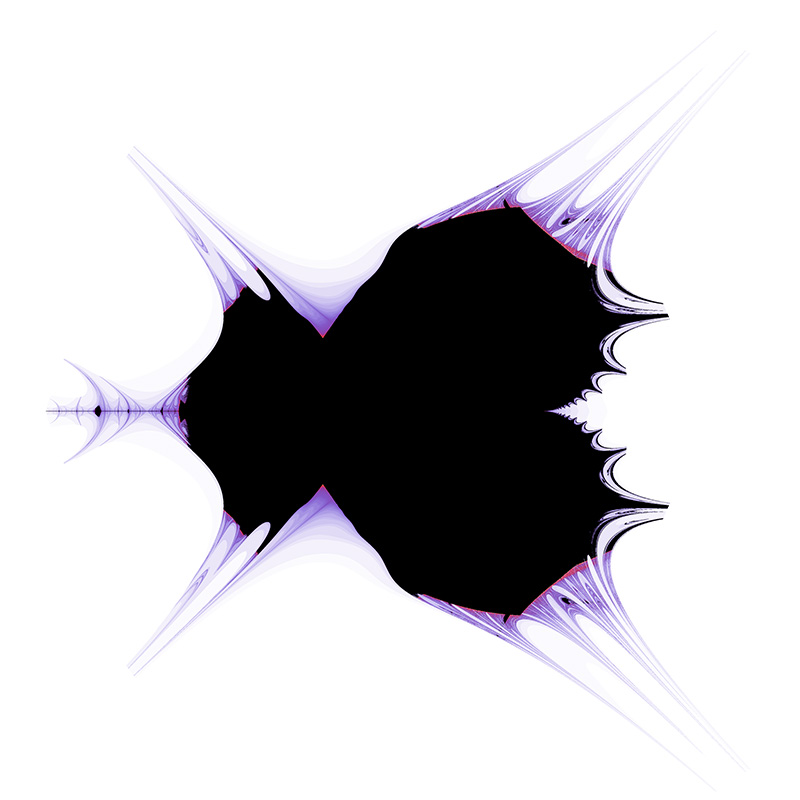# John Tippetts Mandelbrot variation

Written by Paul Bourke
November 2001In 1992 John Tippets published images based upon a variation of the then already famous Mandelbrot set. The Mandelbrot set is an image that describes how the series zn+1 = z2n + c behaves where the complex value c is taken to be each point on the complex plane making up the image. For more information see here. This is normally implemented as follows:

• For each point c = a + bj on the image plane.
• Initialise z0 = x + yj = 0 + 0j
• Loop over the next 4 steps to classify the behaviour of the series
• xnew = x2 - y2 + a
• ynew = 2 x y + b
• x = xnew
• y = ynew

In Tippetts scheme the calculation is as follows. With all due respect to John Tippetts it is hard to imagine this didn't arise from a failed attempt at implementing the real Mandelbrot by a novice programmer.

• For each point c = a + bj on the image plane.
• Initialise z0 = x + yj = 0 + 0j
• Loop over the next 2 steps to classify the behaviour of the series
• x = x2 - y2 + a
• y = 2 x y + b

The overall set is shown below. As with the classical shading of the Mandelbrot, the intensity is proportional to how quickly the series using point c on the plane escapes to infinity.